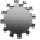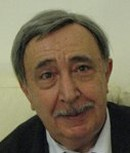Plenary Lecture

Fractional Diffusive Waves Generated by Evolution Equations of Fractional OrderProfessor Francesco Mainardi
Department of Physics
University of Bologna
&
INFN
Italy
E-mail: francesco.mainardi@bo.infn.it

Abstract: In this lecture we discuss some properties of the Cauchy and signaling problems for the one-dimensional time-fractional diffusion-wave equation with the time fractional derivative of order beta (1< beta <2) . In particular, their response to a localized disturbance of the initial data is studied. It is known that whereas the diffusion equation describes a process where the disturbance spreads infinitely fast, the propagation velocity of the disturbance is a constant for the wave equation. We show that the time-fractional diffusion-wave equation interpolates between these two different responses in the sense that the propagation velocities of the maximum points, centers of gravity, and medians of the fundamental solutions to both the Cauchy and the signaling problems are allfinite. On the other hand, the disturbance spreads infinitely fast and the time-fractional diffusion-wave equation is non-relativistic like the classical diffusion equation. Furthermore, the maximum locations, the centers of gravity, and the medians of the fundamental solution to the Cauchy and signaling problems and their propagation velocities are described analytically and calculated numerically. The obtained results for the Cauchy and the signalling problems are interpreted and compared to each other.

Brief Biography of the Speaker: Presently Francesco MAINARDI is retired professor of Mathematical Physics from the University of Bologna where has taught this course since 40 years. His fields of research concern several topics of applied mathematics, including diffusion and wave problems, asymptotic methods, integral transforms, special functions, fractional calculus and non-Gaussian stochastic processes. At present his H-index is > 40 For a full biography, list of references on author's papers and books see: